Courses

# Quantitative Aptitude - Test 1

## 25 Questions MCQ Test SSC CGL Tier 1 Mock Test Series | Quantitative Aptitude - Test 1

Description
This mock test of Quantitative Aptitude - Test 1 for SSC helps you for every SSC entrance exam. This contains 25 Multiple Choice Questions for SSC Quantitative Aptitude - Test 1 (mcq) to study with solutions a complete question bank. The solved questions answers in this Quantitative Aptitude - Test 1 quiz give you a good mix of easy questions and tough questions. SSC students definitely take this Quantitative Aptitude - Test 1 exercise for a better result in the exam. You can find other Quantitative Aptitude - Test 1 extra questions, long questions & short questions for SSC on EduRev as well by searching above.
QUESTION: 1

### A and B can do a piece of work for Rs.800. A alone can do it in 8 days while B alone can do it in 12 days. With help of C, they finish it in 4 days. Find the ratio of share of B and C.

Solution:

From the given data,
A’s one day’s work = 1/8
B’s one day’s work = 1/12
C’s one day’s work = ¼ - (1/8 + 1/12) = 1/24
∴ A : B : C = ratio of their 1 day’s work = 1/8 : 1/12 : 1/24 = 3 : 2 : 1
∴ ratio of share of B and C = 2 : 1

QUESTION: 2

### The curved surface area of a cylindrical pillar is 368 m2 and its volume 1104 m3. Find the height of the cylinder?

Solution:

From the given data
⇒ volume of cylindrical pillar/curved surface area = πr2h/2πrh = 1104/368
⇒ r = (1104 × 2/368) = 6 m
Given that curved surface area = 2πrh = 368
⇒ h = 368/(2π × 6) = 9.76 m
∴ height of the cylinder = 9.76 m

QUESTION: 3

### A wholesaler mixes 23 kg of rice at Rs. 24 per kg with 27 kg of rice of other variety at Rs. 37 per kg and sells the mixture at Rs. 35 per kg. His profit percent is

Solution:

As per the given data,
Cost price of 23 kg of rice = 24 × 23 = Rs.552
Cost price of 27 kg of rice = 27 × 37 = Rs.999
∴ cost price of 50 kg of rice = Rs.552 + Rs.999 = Rs.1551
Also given that he sells at Rs.35 per kg
⇒ selling price of 50 kg of rice = 50 × 35 = 1750
∴ profit percent = (sp - cp/cp × 100)
= (1750 – 1551/1551 × 100)
= 12.83 %

QUESTION: 4

When 30% is lost in grinding, a country can export 60 lakh tons of wheat with some of the wheat still remaining. On the other hand if only 20% is lost in grinding, it can export 80 lakh tons of wheat with the same amount of the wheat remaining, then the production of wheat in the country is

Solution:

From the given data
Let x be the total production
Also from the given data 30% is lost in grinding wheat, a country can export 60 lakh tons of wheat.On the other hand if 20% is lost in grinding,it can export 80 lakh tons of wheat
⇒ 80x/100 – 70x/100 = 80 lakh tons – 60 lakh tons
⇒ 10x/100 = 20 lakh tons
⇒ x = 200 lakhs tons
∴ total production of wheat in the country is 200 lakhs of tons

QUESTION: 5

A train of length 350 m crosses a bridge of length 250 m in 30 seconds. What is speed of the train?

Solution:

As per the given data
Length of the bridge = 250 m
Length of the train = 350 m
Length of the train + length of the bridge = 350 + 250 = 600m
We know that speed = (length of the train + length of bridge)/time
⇒ speed = (600)/30 = 20 m/s = 20 × 18/5 = 72 km/hr
∴ speed of the train = 72km/hr

QUESTION: 6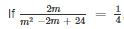,then the least possible value of m is

Solution:

From the given data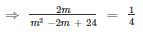⇒ 8m = m2 - 2m + 24
⇒ m2 - 10m + 24 = 0
⇒ m2 - 6m - 4m + 24 = 0
⇒ m(m - 6) - 4(m - 6) = 0
⇒ (m - 4)(m - 6) = 0
⇒ m = 4 and 6
∴ least possible value of m is 4

QUESTION: 7

If α, β are the roots of the equation 2x2 - 3x + 2 = 0, form the equation whose roots are α2, β2 ?

Solution:

As per the given data
⇒ α + β = - b/a = - ( - 3)/2 = 3/2
⇒ αβ = c/a = 2/2 = 1
For the new equation roots are α2 and β2
∴ sum of the roots α2 + β2 = (α + β)2 - 2αβ = (3/2)2 - 2(1) = 9/4 – 2 = ¼
Product of the roots = α2β2 = (αβ)2 = 12 = 1
⇒ Required equation = x2 – (sum of the roots)x + product of the roots = 0
⇒ x2 – (1/4)x + 1 = 0
⇒ 4x2 - x + 4 = 0

QUESTION: 8

The four triangles formed by joining the pairs of mid points of the sides of a given triangle are congruent if the given triangle is

Solution:

It is possible for only an equilateral triangle as all the corresponding sides and all the corresponding angles are equal

QUESTION: 9

In the ΔABC, ∠B = 70° and ∠C = 30°, BO and CO are the angle bisectors of ∠ABC and ∠ACB. Find the value of ∠BOC

Solution:

As per the given data
⇒ ∠B = 70° and ∠C = 30°
∴ ∠OBC = 70/2 = 35° and ∠BCO = 30/2 = 15°
We know that sum of the angles in a triangle = 180°
⇒ ∠BOC + ∠OBC + ∠BCO = 180°
⇒ 35 + 15 + ∠BOC = 180°
⇒ ∠BOC = 180 – 50 = 130°
∴ the value of ∠BOC = 130°

QUESTION: 10

The area of a trapezium is 441 sq.cm and the ratio of parallel sides is 5 : 9. Also the perpendicular distance between them is 21 cm, the longer of parallel sides is

Solution:

From the given data
Let the ratio of parallel sides be 5x : 9x
Also given that perpendicular distance between them h = 21 cm
we know that area of trapezium = ½ (a + b)h
⇒ 441 = ½ (5x + 9x) 21
⇒ 21 = ½ (14x)
⇒ 42 = 14x
⇒ x = 3
Substitute the value of x in 5x and 9x
⇒ 5x = 5(3) = 15 cm
⇒ 9x = 9(3) = 27 cm
∴ longer side of the parallel side = 27 cm

QUESTION: 11

If cos A = 3/5 and sin B = 5/13, find the value of tan (A + B) is

Solution:

As per the given data,
Given that cos A = 3/5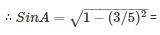4/5 and tan A = SinA/CosA = 4/3
Also given that sin B = 5/13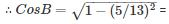12/13 and tan B = SinB/CosB = 5/12
We know that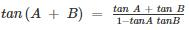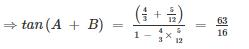QUESTION: 12

When a number is divided by 13, the remainder is 11. When the same number is divided by 17, the remainder is 9. What is the number?

Solution:

From the given data
Let the number be x
We know that dividend = divisor × quotient + remainder
⇒ x = 13p + 11…eq 1
Also given that when the same number is divided by 17, the remainder is 9
⇒ x = 17q + 9...eq 2
From eq 1 and eq 2, we get
⇒ 13p + 11 = 17q + 9
⇒ 17q – 13p = 2
⇒ q = ( 2 + 13p)/17
The least value of p for which q = (2 + 13p)/17 is a whole number is p = 26
Substitute p = 26 in x = 13p + 11
⇒ x = 13× 26 + 11
⇒ x = 349

QUESTION: 13

A shop keeper sells an article at 20% loss. If he sells it for 100 more, he would earn a profit of 10%.The cost price is

Solution:

As per the given data
Let the cost price be x and selling price be y
Given that shopkeeper sells an article at 20% loss
⇒ x – 20x/100 = y
⇒ 80x/100 = y
⇒ x = 5y/4
⇒ 4x - 5y = 0.…eq 1
Also given that if he sells it for 100 more, he would earn a profit of 10%
⇒ x + 10/100 x = y + 100
⇒ 11x = 10y + 1000
⇒ 11x - 10y = 1000.…eq 2
Multiply eq 1 with 2 , we get
⇒ 8x – 10 y = 0 …eq 3
⇒ subtract eq 3 from eq 2 , we get
⇒ 3x = 1000
⇒ x = 333.33
Therefore cost price of the article = Rs.333.33

QUESTION: 14

Average of 100 numbers is 50. If the two numbers 60 and 80 are discarded, then find the average of the remaining numbers ?

Solution:

As per the given data,
Average of 100 numbers = 50
⇒ (x1 + x2 + ….. + x100)/100 = 50
⇒ x1 + x2 + ….. + x100 = 5000
⇒ x1 + x2 + … + x98 = 5000 - 60 - 80
⇒ x1 + x2 + … + x98 = 4860
∴ sum of the 98 numbers = 4860
⇒ average of 98 numbers = 4860/98 = 49.6

QUESTION: 15

The difference between simple interest received from two different sources for Rs.13,000 for 2 years is Rs. 65. Then the difference between their rates of interest?

Solution:

As per the given data
Let their simple interest be I1 and I2
Let their rate of interest be R1 and R2
⇒ I1 – I2 = (PTR1/100 – PTR2/100)
⇒ I1 – I2 = (R1 - R2) (PT/100)
⇒ 65 = (R1 - R2) (13000 × 2/100)
⇒ R1 - R2 = ¼ = 0.25

QUESTION: 16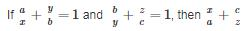will be equal to

Solution: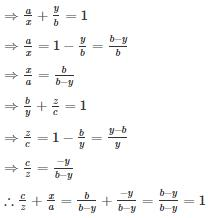QUESTION: 17

If a – b = 3 and a2 + b2 = 29, find the value of a + b ?

Solution:

As per the given data,
⇒ a – b = 3
Squaring on both sides, we get
⇒ a2 + b2 - 2ab = 9
⇒ 29 – 2ab = 9
⇒ 29 – 9 = 2ab
⇒ 20 = 2ab
⇒ ab = 10
We know that (a + b)2 = a2 + b2 + 2ab
⇒ (a + b)2 = 29 + 2(10) = 49
⇒ a + b = √49 = ±7

QUESTION: 18

In ∆ABC, AB = 12 cm, BC = 10 cm and AC = 6 cm. Find the approximate length of the median from vertex A.

Solution:

As per the given data
Let AD be the length of the median subtending from vertex A and to the side BC
⇒ BD = DC = BC/2 = 10/2 = 5 cm
By Apollonius theorem, we get
⇒ AB2 + AC2 = 2 (AD2 + BD2)
⇒ 122 + 6= 2 (AD2 + 52)
⇒ 90 = AD2 + 25
∴ length of the medium = AD = 8.06 ≈ 8 cm

QUESTION: 19

Two arcs of two different circles are of equal lengths. If these arcs subtend angles of 30° and 60° at the centers of their respective circles, find the ratio of the radii of the two circles.

Solution:

As per the given data
Let rand r2 be the radii of the two circles and l be the length of the each arc
We know that l = r θ
⇒ r1θ1 = r2θ2
⇒ r1/r2 = θ21
⇒ r1/r2 = (π/3)/(π/6)
⇒ r1/r2 = 6/3 = 2/1
∴ the required ratio = 2 : 1

QUESTION: 20

The area of a square and circle is same and the perimeter of square and equilateral triangle is same, then the ratio between the area of circle and the area of equilateral triangle is

Solution:

From the given data
Let the each side of a square be a, then its area will be a2
∴ area of circle will also be a2
Also given that the perimeter of square and equilateral triangle is same,
⇒ 4a = 3s
the each side of equilateral triangle is 4a/3
∴ area of equilateral triangle = √3/4 × (4a/3)2 = 4√3 a2/9
∴ ratio between the area of circle and the area of equilateral triangle = a2/(4√3 a2 × 9) = 9 : 4√3

QUESTION: 21

There are two buildings, one on each bank of a river, just opposite to each other. One building is 36 m high. From the top of the this building, the angles of depression of the top and foot of the other building are 30° and 45° respectively. Find the height of the other building?

Solution:

As per the given data,
Let AB and CD be the two buildings, and AC be the river with a length of x meters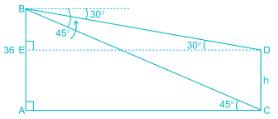Let us consider AB be the building of height = 36 m and CD be the height of other building of h meters
⇒ ∠ACB = 45° and ∠EDB = 30°
From ΔABC, we get
⇒ AB/AC = tan 45°
⇒ 1 = AB/AC
⇒ AB = AC = 36 m
∴ DE = AC = 36 m
From ΔEDB, we get
⇒ tan 30° = BE/DE
⇒ 1/√3 = BE/DE
⇒ BE = DE/√3 = 36/√3 = 20.7 m
We know that CD = AE = AB – BE = (36 - 20.7) m = 15.3 m
∴ height of the other building = 15.3 m

QUESTION: 22

Directions: The pie - chart shows the percentage of expenditure spend by school on different sports. If the amount of total expenditure is Rs. 5,00,000. Study the diagram and answer the following questions.
Expenditure​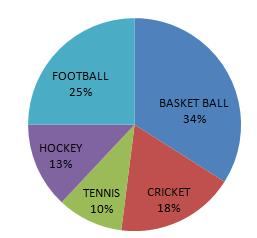Q. ​Find the amount of expenditure that is spend on tennis?

Solution:

As per the given data
Percentage of expenditure that is spend on tennis = 10%
Amount of expenditure that is spend on tennis = 10/100 × 5,00,000 = Rs.50,000

QUESTION: 23

Directions: The pie - chart shows the percentage of expenditure spend by school on different sports. If the amount of total expenditure is Rs. 5,00,000. Study the diagram and answer the following questions.
Expenditure​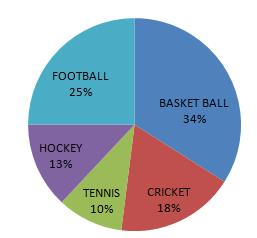Q. ​Find the ratio of expenditure that is spend on cricket and hockey?

Solution:

From the given data
Percentage of expenditure that is spend on cricket = 18%
Amount of expenditure that is spend on cricket = 18/100 × 5,00,000 = Rs. 90,000
Percentage of expenditure that is spend on hockey = 13%
Amount of expenditure that is spend on hockey = 13/100 × 5,00,000 = Rs. 65,000
∴ ratio of expenditure that is spend on cricket and hockey = 90,000 : 65,000 = 18 : 13

QUESTION: 24

Directions: The pie - chart shows the percentage of expenditure spend by school on different sports. If the amount of total expenditure is Rs. 5,00,000. Study the diagram and answer the following questions.
Expenditure​Q. How much expenditure more is spent on basketball than football?

Solution:

From the given data
Percentage of expenditure that is spend on basketball = 34%
Amount of expenditure that is spend on basketball = 34/100 × 5,00,000 = Rs. 1,70,000
Percentage of expenditure that is spend on football = 25%
Amount of expenditure that is spend on football = 25/100 × 5,00,000 = Rs. 1,25,000
Difference of expenditure that is spend on basketball and football = 170000 - 125000 = Rs. 45,000
∴ Amount of Rs. 45,000 more is spend on basketball than football

QUESTION: 25

Directions: The pie - chart shows the percentage of expenditure spend by school on different sports. If the amount of total expenditure is Rs. 5,00,000. Study the diagram and answer the following questions.
Expenditure​Q. Find the ratio of expenditure on football to the total expenditure?

Solution:

From the given data
Total expenditure spend on all the sports = Rs. 5,00,000
Percentage of expenditure that is spend on football = 25%
Amount of expenditure that is spend on football = 25/100 × 5,00,000 = Rs. 1,25,000
∴ ratio of expenditure that is spend on football to the total expenditure = 1,25,000 : 5,00,000 = 1 : 4